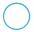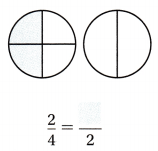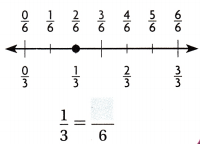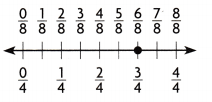Refer to our Texas Go Math Grade 3 Answer Key Pdf to score good marks in the exams. Test yourself by practicing the problems from Texas Go Math Grade 3 Module 3 Assessment Answer Key.

Vocabulary

Choose the best term from the box to complete the sentence.

Question 1.
___ are two or more fractions that name the same amount. (p. 87)

Concepts and Skills

Question 2.
When two fractions refer to the same whole and have the same denominators, explain why you can compare only
the numerators. TEKS 3.3.A, 3.3.H

Use models to compare. Write <, >, or =. TEKS 3.3A, 3.3.H

Question 3.
$$\frac{1}{6}$$$$\frac{1}{4}$$

Question 4.
$$\frac{1}{8}$$$$\frac{1}{8}$$

Question 5.
$$\frac{2}{8}$$$$\frac{2}{3}$$

Question 6.
$$\frac{4}{2}$$$$\frac{1}{2}$$

Question 7.
$$\frac{7}{8}$$$$\frac{3}{8}$$

Question 8.
$$\frac{6}{6}$$$$\frac{6}{8}$$

Shade the model or use the number line to find the equivalent fraction.

Question 9.Question 10.Question 11.Texas Test Prep

Fill in the bubble for the correct answer choice.

Question 12.
Two walls in Tiffany’s room are the same size. Tiffany paints $$\frac{1}{4}$$ of one wall. Jake paints $$\frac{1}{8}$$ of the other wall. Which of the following correctly compares the fractions? TEKS 3.3.A, 3.3.H
(A) $$\frac{1}{4}$$ < $$\frac{1}{8}$$
(B) $$\frac{1}{8}$$ = $$\frac{1}{4}$$
(C) $$\frac{1}{4}$$ > $$\frac{1}{8}$$
(D) $$\frac{1}{8}$$ > $$\frac{1}{4}$$

Question 13.
Matthew divided a banana into sixths and ate two parts. What is an equivalent fraction for $$\frac{2}{6}$$? TEKS 3.3.A, 3.3.F, 3.3.G(A) $$\frac{2}{3}$$
(B) $$\frac{1}{2}$$
(C) $$\frac{1}{4}$$
(D) $$\frac{1}{3}$$

Question 14.
Gabby cut an apple into 2 equal pieces and ate 1 of the pieces. What is an equivalent fraction for the portion of apple Gabby ate? TEKS 3.3.A, 3.3.G(A) $$\frac{3}{4}$$
(B) $$\frac{4}{8}$$
(C) $$\frac{2}{8}$$
(D) $$\frac{3}{8}$$
Liam rowed his boat $$\frac{3}{4}$$ mile across the lake. What is an equivalent fraction for $$\frac{3}{4}$$? TEKS 3.3.A, 3.3.B, 3.3.F, 3.3.G(A) $$\frac{2}{3}$$
(B) $$\frac{3}{6}$$
(C) $$\frac{4}{8}$$
(D) $$\frac{6}{8}$$